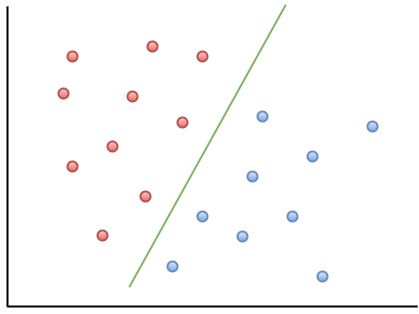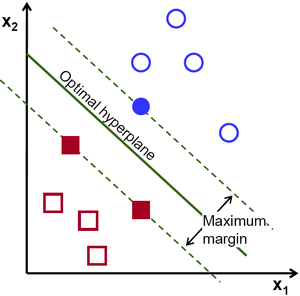# Introduction to Support Vector Machines

Hey - Nick here! This page is a free excerpt from my new eBook Pragmatic Machine Learning, which teaches you real-world machine learning techniques by guiding you through 9 projects.

Since you're reading my blog, I want to offer you a discount. Click here to buy the book for 70% off now.

In this tutorial, you will receive your first introduction to support vector machines.

We will continue our discussion of support vector machines by learning to code Python support vector machines in the next tutorial.

## Table of Contents

You can skip to a specific section of this Python machine learning tutorial using the table of contents below:

## What Are Support Vector Machines?

Support vector machines - or SVMs for short - are supervised machine learning models with associated learning algorithms that analyze data and recognize patterns.

Support vector machines can be used for both classification problems and regression problems. In this course, we will specifically be looking at the use of support vector machines for solving classification problems.

## How Do Support Vector Machines Work?

Let's dig in to how support vector machines really work.

Given a set of training examples, each marked for belonging to one of two categories, a support vector machine training algorithm builds a model that assigns new examples into one of the two categories. This makes the support vector machine a non-probabilistic binary linear classifier.

The SVM uses geometry to make categorical predictions.

More specifically, an SVM model maps the data points as points in space and divides the separate categories so that they are divided by an open gap that is as wide as possible. New data points are predicted to belong to a category based on which side of the gap they belong to.

Here is an example visualization that can help you understand the intuition behind support vector machines:As you can see, if a new data point falls on the left side of the green line, it will be labeled with the red category. Similarly, if a new data point falls on the right side of the green line, it will get labelled as belonging to the blue category.

This green line is called a `hyperplane`, which is an important piece of vocabulary for support vector machine algorithms.

Let's take a look at a different visual representation of a support vector machine:In this diagram, the `hyperplane` is labelled as the `optimal hyperplane`. Support vector machine theory defines the `optimal hyperplane` as the one that maximizes the margin between the closest data points from each category.

As you can see, the margin line actually touches three data points - two from the red category and one from the blue category. These data points which touch the margin lines are called `support vectors` and are where support vector machines get their name from!

## Final Thoughts

In this tutorial, you received your first introduction to support vector machine models.

Here is a brief summary of what we discussed in this tutorial:

• That support vector machines are an example of a supervised machine learning algorithm
• That support vector machines can be used to solve both classification and regression problems
• How support vector machines categorize data points using a `hyperplane` that maximizes the margin between categories in a data set
• That the data points that touch margin lines in a support vector machine are called `support vectors`. These data points are where support vector machines derive their name from